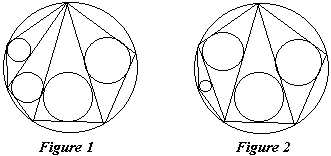R. Honsberger,
Mathematical Gems, III,
MAA, 1985, pp. 24-26

## An Old Japanese Theorem

In Roger Johnson's marvellous old geometry text-Advanced Euclidean Geometry, first published in 1929 - he reports (on page 193 of the Dover edition, 1960) on the ancient custom by Japanese mathematicians of inscribing their discoveries on tablets which were hung in the temples to the glory of the gods and the honor of the authors. The following gem is known to have been exhibited in this way in the year 1800.

Let a convex polygon, which is inscribed in a circle, be triangulated by drawing all the diagonals from one of the vertices, and let the inscribed circle be drawn in each of the triangles. Then the sum of the radii of all these circles is a constant which is independent of which vertex is used to form the triangulation (Figure 1).

A great deal more might have been claimed, for this same sum results for every way of triangulating the polygon! (Figure 2). As we shall see, a simple application of a beautiful theorem of L. N. M. Carnot (1753-1823) settles the whole affair.First of all, observe that any triangulation of an n-gon with its diagonals consists of (n - 2) triangles. Next assume that the triangles in the triangulation are numbered, and that the inradius of triangle i is ri; moreover, denote the sum of distances in the Carnot's theorem as OOi. Then, observing that every triangle in the triangulation has the given circle as its circumcircle, Carnot's thereom states

ri + R = OOi

and the sum in question is given by

 (*) r1 + r2 + ... + rn-2 = OO1 + OO2 + ... + OOn-2 - (n - 2)RNote now that the sum OO1 + OO2 + ... + OOn-2 on the right consists of perpendiculars to the sides of the polygon each counted once, and perpendiculars to the diagonals each counted twice since every diagonal serves as a side of two adjacent triangles. Furthermore, considering such internal perpendiculars, each is taken once with the sign plus and another time with the sign minus thus contributing nothing to the sum. Therefore, this sum is actually equal to the sum of all perpendiculars from O to the sides of the polygon. And, therefore, the right-hand side in (*) is constant. So is the left-hand side.

### Remark 1

There is another theorem that is also known as Japanese.

### Remark 2

David Richeson of Dickinson College has extended (2011) the theorem to non-convex polygons.### References

1. R. Honsberger, Mathematical Gems III, MAA, 1985
2. R. A. Johnson, Advanced Euclidean Geometry (Modern Geometry), Dover, 1960## Sangaku